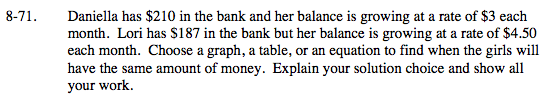### Home > MC2 > Chapter 8 > Lesson 8.2.4 > Problem8-71

8-71.To find the amount of money Daniella has gained, multiply the rate (\$3) by the number of months.

To find the total amount of money Daniella has in the bank, the equation would be 210 + 3x, where x represents the number of months that have passed.
Find the equation that represents the amount of money Lori has in the bank.

Since you are looking for the time at which the girls will have the same amount of money, set the two equations equal to each other.

210 + 3x = 187 + 4.5x
Solve for x.

$\text{15\frac{1}{3} months}$